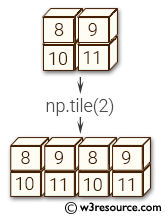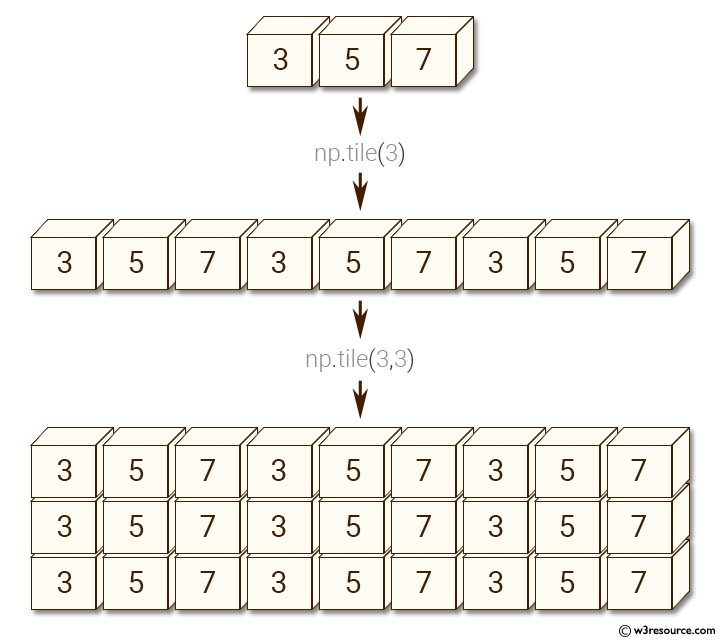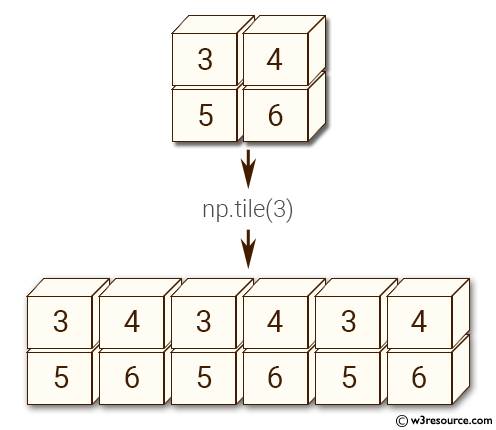# NumPy Array manipulation: tile() function

## numpy.tile() function

The tile() function is used to construct an array by repeating A the number of times given by reps.
If reps has length d, the result will have dimension of max(d, A.ndim).
If A.ndim < d, A is promoted to be d-dimensional by prepending new axes. So a shape (3,) array is promoted to (1, 3) for 2-D replication, or shape (1, 1, 3) for 3-D replication. If this is not the desired behavior, promote A to d-dimensions manually before calling this function.
If A.ndim > d, reps is promoted to A.ndim by pre-pending 1’s to it. Thus for an A of shape (2, 3, 4, 5), a reps of (2, 2) is treated as (1, 1, 2, 2).

Note: Although tile may be used for broadcasting, it is strongly recommended to use numpy’s broadcasting operations and functions

Syntax:

`numpy.tile(A, reps)`Version: 1.15.0

Parameter:

Name Description Required /
Optional
a The input array. Required
reps The number of repetitions of A along each axis. Required

Return value:

c [ndarray] The tiled output array.

Example-1: numpy.tile() function

``````>>> import numpy as np
>>> x = np.array([3, 5, 7])
>>> np.tile(x, 3)
array([3, 5, 7, 3, 5, 7, 3, 5, 7])
>>> np.tile(x, (3,3))
array([[3, 5, 7, 3, 5, 7, 3, 5, 7],
[3, 5, 7, 3, 5, 7, 3, 5, 7],
[3, 5, 7, 3, 5, 7, 3, 5, 7]])
``````

Pictorial Presentation:Example-2: numpy.tile() function

``````>>> import numpy as np
>>> x = np.array([3, 5, 7])
>>> np.tile(x, (3, 1, 3))
array([[[3, 5, 7, 3, 5, 7, 3, 5, 7]],

[[3, 5, 7, 3, 5, 7, 3, 5, 7]],

[[3, 5, 7, 3, 5, 7, 3, 5, 7]]])
``````

Example-3: numpy.tile() function

``````>>> import numpy as np
>>> y = np.array([[3, 4], [5, 6]])
>>> np.tile(y, 3)
array([[3, 4, 3, 4, 3, 4],
[5, 6, 5, 6, 5, 6]])
``````

Pictorial Presentation:Example-4: numpy.tile() function

``````>>> import numpy as mp
>>> np.tile(y, (3, 1))
array([[3, 4],
[5, 6],
[3, 4],
[5, 6],
[3, 4],
[5, 6]])
>>> z = np.array([3, 5, 7, 9])
>>> np.tile(z,(4,1))
array([[3, 5, 7, 9],
[3, 5, 7, 9],
[3, 5, 7, 9],
[3, 5, 7, 9]])
``````

Python - NumPy Code Editor:

Previous: vsplit()
Next: repeat()

﻿